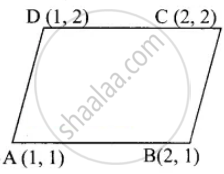Tamil Nadu Board of Secondary EducationSSLC (English Medium) Class 9th
Advertisement Remove all ads

# Verify that the following points taken in order to form the vertices of a rhombus A(1, 1), B(2, 1), C(2, 2) and D(1, 2) - Mathematics

Sum

Verify that the following points taken in order to form the vertices of a rhombus

A(1, 1), B(2, 1), C(2, 2) and D(1, 2)

Advertisement Remove all ads

#### SolutionDistance = sqrt((x_2 - x_1)^2 + (y_2 - y_1)^2

AB = sqrt((2 - 1)^2 + (1 - 1)^2

= sqrt((1)^2 + (0)^2

= sqrt(1)

= 1

BC = sqrt((2 - 2)^2 + (2 - 1)^2

= sqrt((0)^2 + (1)^2

= sqrt(1)

= 1

CD = sqrt((1 - 2)^2 + (2 - 2)^2

= sqrt((- 1)^2 + (0)^2

= sqrt(1)

= 1

AD = sqrt((1 - 1)^2 + (2 - 1)^2

= sqrt((0)^2 + (1)^2

= sqrt(1)

= 1

AB = BC = CD = AD = 1.

All the four sides are equal.

∴ ABCD is a rhombus.

Concept: Co-ordinates of Points and Distance
Is there an error in this question or solution?
Advertisement Remove all ads

#### APPEARS IN

Samacheer Kalvi Mathematics Class 9th Tamil Nadu State Board
Chapter 5 Coordinate Geometry
Exercise 5.2 | Q 6. (ii) | Page 203
Advertisement Remove all ads
Advertisement Remove all ads
Share
Notifications

View all notifications

Forgot password?